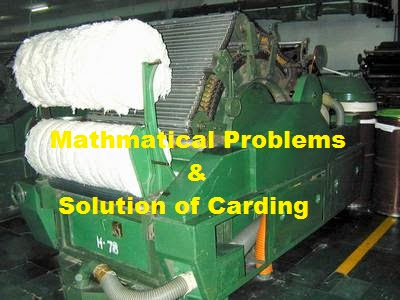# Textile Calculation

Problems and solutions of textile

## Mathematical Problems and Solutions of Cotton Carding Section

Mathematical Problems and Solutions of Cotton Carding SectionMd. Abu Sayed
Founder of Textile Apex
Pabna Textile engineering college, Pabna, Bangladesh
Cell : +8801745214773

Carding Section:
The first condition of spinning is to open the fiber at single stage and which is possible only by carding. The quality of yarn specially depends on the carding action, so carding is called the heart of spinning. Here Calculation plays a vital role. So we need to know about the carding calculations.Problems and Solutions of Cotton Carding:

1. Draft constant of a carding m/c is 2194 and if the DCP is 22T, then what will be the draft of the m/c?

Solution:
Here,
• Draft constant of a carding m/c = 2194
• DCP of carding = 22T
• Draft of carding m/c =?
We know that,

Draft constant
Draft = ………………. = 2194/22 = 99.72 (ANS)
DCP

2. If the draft of a carding m/c is 110 and DCP is 20T, then what will be the draft constant?

Solution:
Here,
• Carding m/c draft = 110
• Carding m/c DCP = 20T
• Draft constant of carding m/c =?
We know that,

Draft constant = Draft X DCP = 110 X 20 = 2200 (ANS).

3. If a carding m/c produces 64 grain/gauge sliver by 19T DCP, then what is the DCP needed to produce 70 grain/gauge wt. sliver?

Solution:
Here given,
• Present DCP = 19T
• Wt. of present sliver = 64 grain/gauge
• Wt. of new sliver = 70 grain/gauge
• New DCP =?
We know that,

Present DCP X Wt. of new sliver
New DCP = ………………………………………
Wt. of present sliver

= (19 X 70)/64 = 20.78 = 21T (ANS)

4. Calculate the new sliver hank from the following data –
• Present hank = 0.16
• Present DCP = 18
• New DCP = 24
Solution:
Here given,
• Present hank = 0.16
• Present DCP = 18
• New DCP = 24
• New sliver hank =?
We know that,

Present DCP
New sliver hank = Present sliver hank X ………………
New DCP

= 0.16 X 18/24 = 0.12 (ANS).

5. A carding m/c produces 0.17 hank slivers from 13 oz (ounce)/gauge wt. lap by wasting 6%. What will be the mechanical draft of the machine?
Solution:
Here given,
• Waste = 6%
• Feeded lap wt. = 13 oz/gauge
• Carded sliver hank = 0.17
• Mechanical draft =?
Lap length (gauge)                1 lb
Lap hank = ……………………… X …………………
840                          Lap wt. (lb)

1               1                      16
= ……….. X …………. = …………… = 0.00146
840           13/16            840 X 13

Again,

Actual draft of carding m/c = Carded sliver hank / Lap hank

= 0.17/0.00146 = 116.44

Mechanical draft of carding m/c = Actual draft X (100 –Waste) / 100

= 116.44 X (100 – 6) / 100 = 109.45 (ANS).

6. If a carding m/c produces 70 grain/gauge sliver from 14 oz/gauge wt. lap. Then what will be the actual draft of the carding m/c?

Solution:
Here given,
• Feed lap wt. = 14 oz/gauge
• Wt. of delivered sliver = 70 grain/gauge
• Actual draft =?
Lap wt. in oz
Lap wt. in grain = ……………… X 7000
16

= 14/16 X 7000             (1 lb = 7000 grain)

= 6125

Actual draft = Feeded lap wt. / Delivered sliver wt.

= 6125/70 = 87.5 (ANS)

7. In a carding m/c, rotation of doffer 15 rpm, doffer circumference is 85 inch, Wt. of produced sliver 65 grain/gauge and efficiency is 85%. What is the production per day of the carding m/c (in metric ton)?

Solution:
Here given,
• Rotation of doffer = 15 rpm
• Doffer circumference = 85 inch
• Wt. of produced sliver = 65 grain/gauge
• Eff. Of carding m/c = 85%
• Production per day =?
We know,

Production = Doffer circumference X Speed X Eff.%

= 85 X 15 X 85/100 inch/min

= 85 X 15 X (60 X 24)/36 X 65 X 85/100 grain/day

(As, 7000 grain = 1 lb and 2204 lb = 1 metric ton)

= 85 X 15 X (60 X 24)/36 X 65 X 1/ (7000 X 2204) X 85/100 Metric ton

= 0.183 Metric ton/day (ANS).

8. In a carding m/c rotation speed of calendar roller 80/min, Dia of calendar roller is 4”, Produced sliver hank is 0.14, Eff. Of carding m/c is 85%, If the tension draft of the m/c is 1.05 than calculate the production per shift (8 hr) of the carding m/c?

Solution:
From the given data,
• Rotation speed of calendar roller = 80/min
• Dia of calendar roller = 4 inch
• Produced sliver hank = 0.14
• Eff. Of carding m/c = 85%
• Tension draft of carding m/c = 1.05
• Production/hr =?
We know that,

Production / shift = Circumference of calendar roller X (60 X 8) / (36 X 840 X Hank)
X Tension draft X Eff.%

= 3.14 X 4 X 80 X (60 X 8)/(36 X 840 X 0.14) X 1.05 X 85/100

= 101.68 lb (ANS).